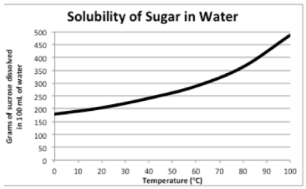# Problem: Using the graph, what is the solubility of sugar in water at 90°C?Knowing that sugar is essentially sucrose (342.3 g/mol), determine the value of K sp for sugar in water at 90°C.

###### FREE Expert Solution

Knowing that sugar is essentially sucrose (342.3 g/mol), determine the value of K sp for sugar in water at 90°C.

We are being asked for the solubility using the graph and the Ksp of sucrose.

• To determine the solubility, we need to draw a vertical line from 90o###### Problem Details

Using the graph, what is the solubility of sugar in water at 90°C?

Knowing that sugar is essentially sucrose (342.3 g/mol), determine the value of K sp for sugar in water at 90°C.